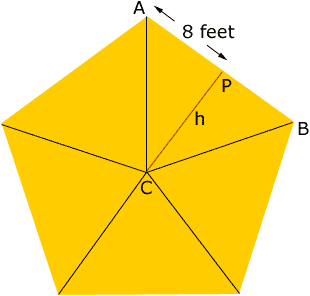Quandaries and Queries Hi, My name is Susan. My problem is this: I need to calculate the square footage of a regular pentagon (all angles are equal).  Each side is 16 feet long.  Can you help me with this? Thank you. Hi Susan, Join each vertex of the pentagon to the centre C to form five congruent triangles. Label two adjacent vertices A and B and let P be the midpoint of AB. The length of the line PC is then the height h of the triangle ACB.Since AB is 16 feet long, AP is 8 feet long. Since the five triangles are congruent the angle ACB is one-fifth of 360o which is 720 and hence angle ACP is 360. Thus tan( 360) = 8/h so 0.7265h = 8 and h = 11.01 feet. Thus the area of the triangle ACB is 811.01 square feet and hence the area of the pentagon is 5811.01 = 440.4 square feet. Penny Go to Math Central